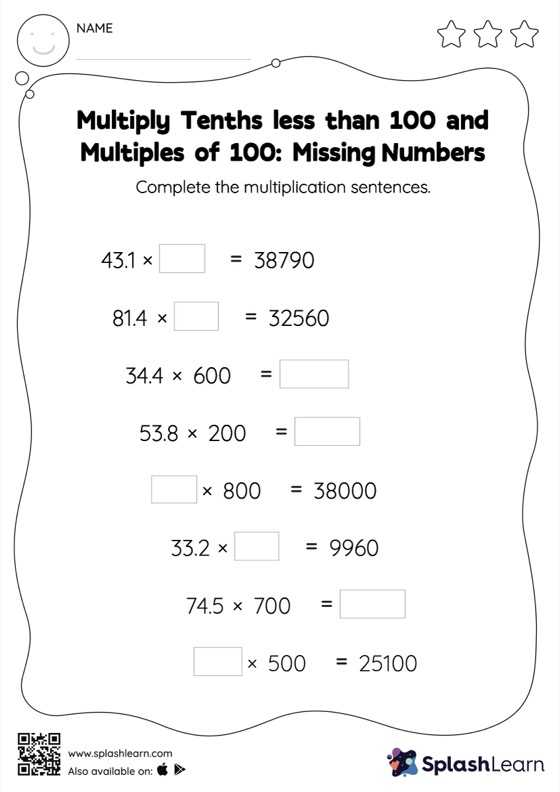# Multiply Tenths less than 100 and Multiples of 100: Missing Numbers Worksheet

Home > Multiply Tenths less than 100 and Multiples of 100: Missing NumbersCan your young mathematician multiply tenths less than 100 and multiples of 100? That's exactly what they do here. As the worksheet uses a horizontal format, it allows for more creativity when it comes to how the student solves the problem. In contrast, in the vertical format, students usually employ the standard method to solve.